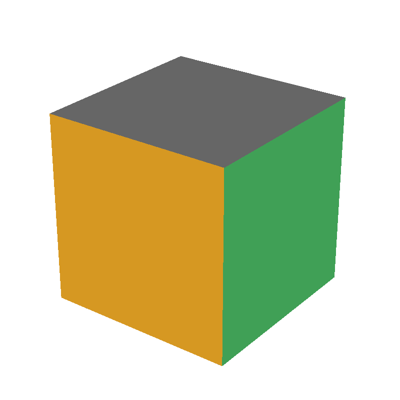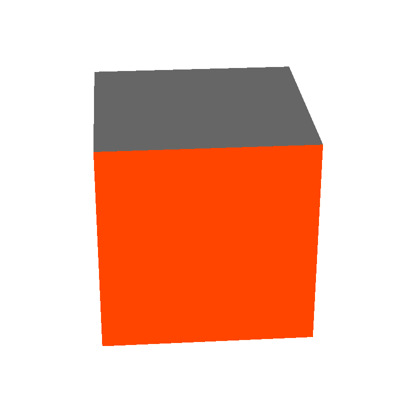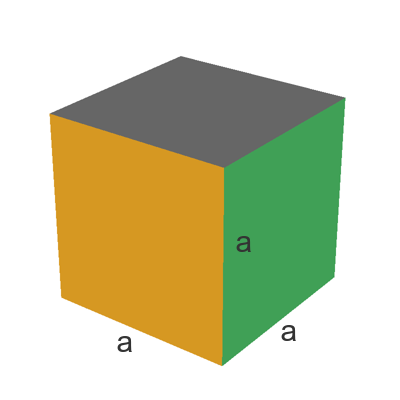# Cube

Martin McBride
2021-02-11

A cube is a square box (or block) shape:The faces of a cube are all square.

Here is a rotating view of a cube:## Cube facts

A cube has 6 faces - the top, bottom, and 4 sides.

It has 8 corners (vertices).

It has 12 edges.

## Cube volume

Here is a cube of side $a$:The width, height and length of the cube are all equal to $a$.

This means that the volume is:

$$volume = a \times a \times a = a^3$$

The volume is $a$ cubed (this is where the term cubed comes from).

Each of the 6 faces of the cube is a square of size $a$ by $a$.